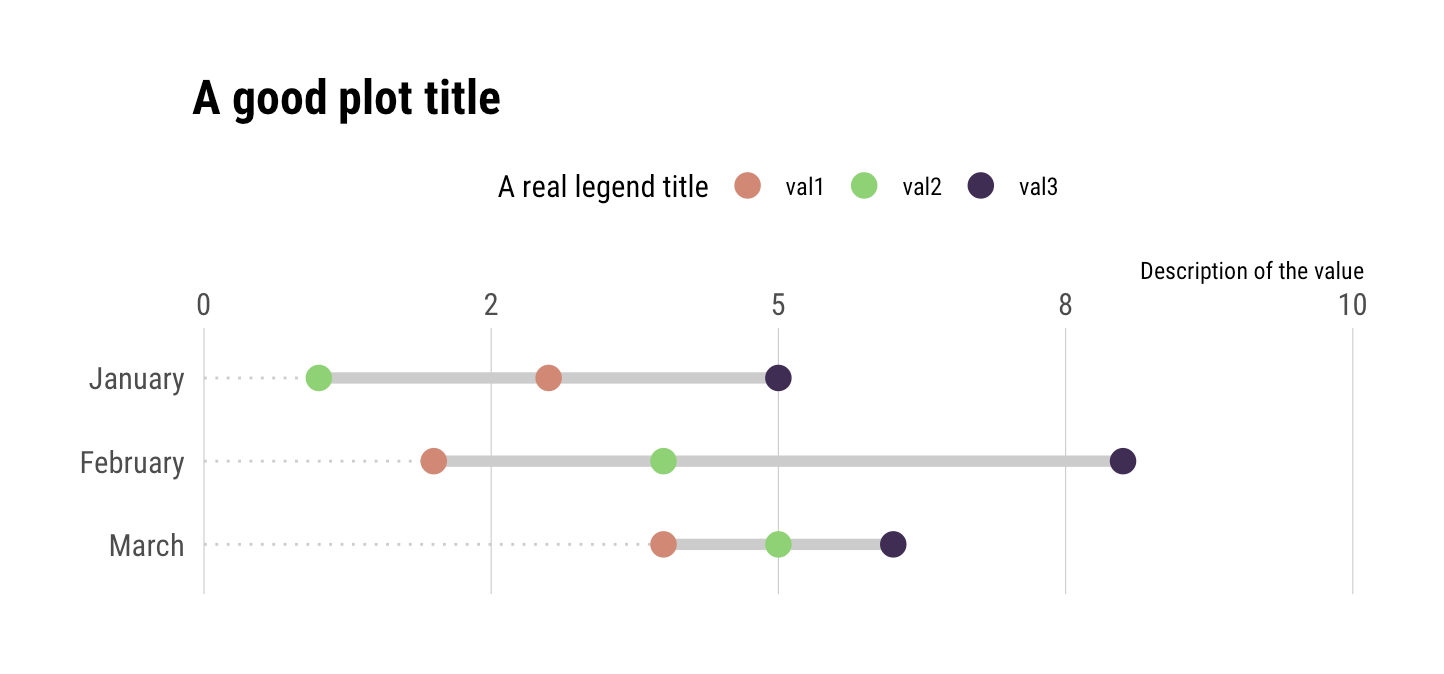# Make Multi-point “dumbbell” Plots in ggplot2

A user of the `{ggalt}` package recently posted a question about how to add points to a `geom_dumbbell()` plot. For now, this is not something you can do with `geom_dumbbell()` but with a bit of data wrangling you can do this in a pretty straightforward manner with just your data and ggplot2. The example below uses 3 values per category but it should scale to `n` values per category (though after a certain `n` you should reconsider the use of a dummbell chart in favour of a more appropriate way to visualize the message you’re trying to convey).

Here’s the setup:

``library(hrbrthemes)library(tidyverse)tibble(  val1 = c(3, 2, 4),  val2 = c(1, 4, 5),  val3 = c(5, 8, 6),  cat = factor(month.name[1:3], levels = rev(month.name[1:3]))) -> xdf``

Three values per category. The approach is pretty straightforward:

• reshape the data frame & get min value so you can draw an eye-tracking line (this is one geom)
• reshape the data frame & get min/max category values so you can draw the segment (this is another geom)
• reshape the data frame & plot the points

I’ve put ^^ notes near each ggplot2 geom:

``ggplot() +  # reshape the data frame & get min value so you can draw an eye-tracking line (this is one geom)  geom_segment(    data = gather(xdf, measure, val, -cat) %>%       group_by(cat) %>%       top_n(-1) %>%       slice(1) %>%      ungroup(),    aes(x = 0, xend = val, y = cat, yend = cat),    linetype = "dotted", size = 0.5, color = "gray80"  ) +  # reshape the data frame & get min/max category values so you can draw the segment (this is another geom)  geom_segment(    data = gather(xdf, measure, val, -cat) %>%       group_by(cat) %>%       summarise(start = range(val), end = range(val)) %>%       ungroup(),    aes(x = start, xend = end, y = cat, yend = cat),    color = "gray80", size = 2  ) +  # reshape the data frame & plot the points  geom_point(    data = gather(xdf, measure, value, -cat),    aes(value, cat, color = measure),     size = 4  ) +  # i just extended the scale a bit + put axis on top; choose aesthetics that work   # for you  scale_x_comma(position = "top", limits = c(0, 10)) +  scale_color_ipsum(name = "A real legend title") +  labs(    x = "Description of the value", y = NULL,    title = "A good plot title"  ) +  theme_ipsum_rc(grid = "X") +  theme(legend.position = "top")``

And, here’s the result:Recent Articles By Author*** This is a Security Bloggers Network syndicated blog from rud.is authored by hrbrmstr. Read the original post at: https://rud.is/b/2019/06/06/make-multi-point-dumbbell-plots-in-ggplot2/